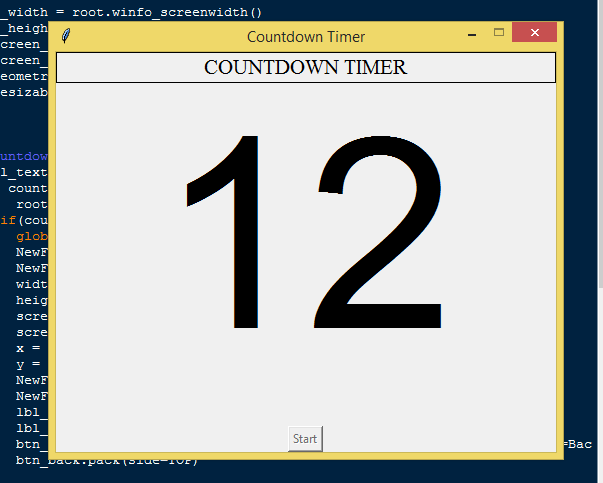# Introduction

In this tutorial we will create a How to Create a Countdown Timer in Python. This tutorial purpose is to provide a countdown timer for triggering any event. This will cover all the basic parts for creating the countdown timer. I will provide a sample program to show the actual coding of this tutorial.

This tutorial will make it easy for you to understand the coding just follow my instruction I provided and you can do it without a problem. This program is very useful when you want to trigger any event that needed timer. I will try my best to give you the easiest way of creating this program Countdown Timer in python. So let's do the coding.

## Importing Modules

After setting up the installation and the database, run the IDLE and click file and then new file. After that a new window will appear containing a black file this will be the text editor for the python. Then copy code that I provided below and paste it inside the IDLE text editor.
`from tkinter import *`

## Setting up the Main Frame

After importing the modules, we will now then create the main frame for the application. To do that just copy the code below and paste it inside the IDLE text editor.
`root = Tk()root.title("Countdown Timer")width = 500height = 400screen_width = root.winfo_screenwidth()screen_height = root.winfo_screenheight()x = (screen_width/2) - (width/2)y = (screen_height/2) - (height/2)root.geometry("%dx%d+%d+%d" % (width, height, x, y))root.resizable(0, 0)`

## Creating Main Function

This is the main function of the application is. This code will create a timer that can be trigger with a certain argument. To do this just copy and write these block of codes inside the IDLE text editor.
`def countdown(count):    lbl_text['text'] = count    if count > 0:        root.after(1000, countdown, count-1)    elif(count == 0):        global NewForm        NewForm = Toplevel()         NewForm.title("Countdown Timer")        width = 500        height = 300        screen_width = root.winfo_screenwidth()        screen_height = root.winfo_screenheight()        x = (screen_width/2) - (width/2)        y = (screen_height/2) - (height/2)        NewForm.geometry("%dx%d+%d+%d" % (width, height, x, y))        NewForm.resizable(0, 0)        lbl_blast = Label(NewForm, text="TIME EXPIRED!", font=('arial', 50))        lbl_blast.pack(fill=BOTH, pady=100)        btn_back = Button(NewForm, text="Reset", font=('arial', 16), command=BackBtn)        btn_back.pack(side=TOP)  def StartCountdown():    countdown(20)    btn_toggle.config(state=DISABLED)  def BackBtn():    NewForm.destroy()    btn_toggle.config(state=NORMAL)    lbl_text.config(text="20")`

In the given code we first create a method called countdown(), it will start the countdown process using the after() function. Then we create a method that will trigger the countdown() function to start the timer called StartCountdown().

## Designing the Layout

After creating the Functions we will now add some layout to the application. Just kindly copy the code below and paste it inside the IDLE text editor.
`Top = Frame(root, width=500, bd=1, relief=SOLID)Top.pack(side=TOP)TextField = Frame(root)TextField.pack(side=TOP)BtnGroup = Frame(root, width=500)BtnGroup.pack(side=BOTTOM) lbl_title = Label(Top, width=500, text="COUNTDOWN TIMER", font=('times new roman', 16))lbl_title.pack(fill=X)lbl_text = Label(TextField, text="20", font=('arial', 200))lbl_text.pack(fill=BOTH)  btn_toggle = Button(BtnGroup, text="Start", command=StartCountdown)btn_toggle.pack()`

## Initializing the Application

After finishing the function save the application as index.py. This function will run the code and check if the main is initialize properly. To do that copy and write these block code after the main function inside the IDLE text editor.

`if __name__ == '__main__':    root.mainloop()`

## Output: